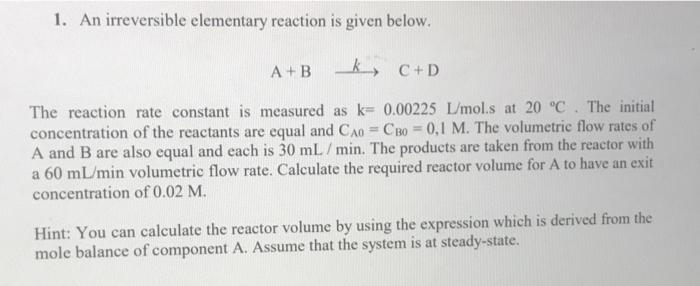# Question Solved1 AnswerCSTR REACTOR 1. An irreversible elementary reaction is given below. A+ Bk, C+D The reaction rate constant is measured as k= 0.00225 L/mol.s at 20 °C. The initial concentration of the reactants are equal and CA = CB0 = 0,1 M. The volumetric flow rates of A and B are also equal and each is 30 mL/min. The products are taken from the reactor with a 60 mL/min volumetric flow rate. Calculate the required reactor volume for A to have an exit concentration of 0.02 M. Hint: You can calculate the reactor volume by using the expression which is derived from the mole balance of component A. Assume that the system is at steady-state.4OXUNE The Asker · Chemical EngineeringCSTR REACTOR
Transcribed Image Text: 1. An irreversible elementary reaction is given below. A+ Bk, C+D The reaction rate constant is measured as k= 0.00225 L/mol.s at 20 °C. The initial concentration of the reactants are equal and CA = CB0 = 0,1 M. The volumetric flow rates of A and B are also equal and each is 30 mL/min. The products are taken from the reactor with a 60 mL/min volumetric flow rate. Calculate the required reactor volume for A to have an exit concentration of 0.02 M. Hint: You can calculate the reactor volume by using the expression which is derived from the mole balance of component A. Assume that the system is at steady-state.
More
Transcribed Image Text: 1. An irreversible elementary reaction is given below. A+ Bk, C+D The reaction rate constant is measured as k= 0.00225 L/mol.s at 20 °C. The initial concentration of the reactants are equal and CA = CB0 = 0,1 M. The volumetric flow rates of A and B are also equal and each is 30 mL/min. The products are taken from the reactor with a 60 mL/min volumetric flow rate. Calculate the required reactor volume for A to have an exit concentration of 0.02 M. Hint: You can calculate the reactor volume by using the expression which is derived from the mole balance of component A. Assume that the system is at steady-state.